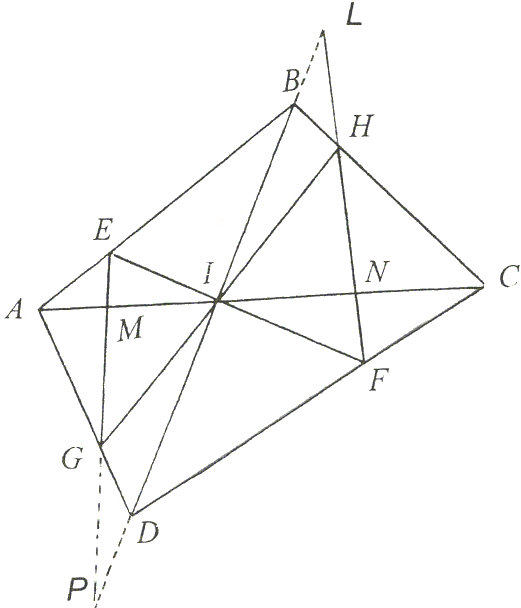## The Lepidoptera of the Quadrilateral II

### Sidney Kung April 26, 2008

We prove the Butterfly Theorem with the aid of Menelaus' theorem.

Through the intersection I of the diagonals AC, BD of a convex quadrilateral ABCD, draw two lines EF and HG that meet the sides of ABCD in E, F, G, H. Let M and N be the intersections of EG and FH with AC. Then

1/IM - 1/IA = 1/IN - 1/IC.

### ProofFigure 1.

Refer to Figure 1. Triangles BAD and CAD are cut by transversal EIF. So, by applying Menelaus' theorem twice, we have

BE/EA · AK/KD · DI/IB = 1,
CF/FD · DK/KA · AI/IC = 1.

Multiplying the above gives

BE/EA · ID/IB · CF/FD · IA/IC = 1,

or

 (1) IA · ID · BE/EA = IC · IB · FD/CF.

Similarly, since transversal HIG cuts triangles BDC and ADC, applying Menelaus' theorem twice again will give us

AG/GD · DI/IB · BH/HC · CI/IA = 1,

or

 (2) ID · IC · BH/HC = IB · IA · DG/GA.

Note that neither (1) nor (2) involves IM, MA, IN, or NC. The relationship among these and other line segments in the figure will be established as follows:Figure 2.

Refer to Figure 2. Let L and P be the intersections of FH and EG with BG. Consider the triangle CDI with transversal FH, we have

DF/ FC · CN/NI · IL/LD =1,

or

 (3) DF/FC = IN/NC · LD/IL.

Similarly, from triangle CIB, we see that

BH/HC · CN/NI · IL/LB = 1,

or

 (4) BH/HC = NI/CN · LB/IL.

Thus,

(5)
 IB · DF/ FC + DI · BH/HC = IB · IN/NC · LD/IL + DI · NI/CN · LB / IL = NI/CN · (IB · ID + DI · LB) / IL = NI/CN · (IB · (DI + IL) + DI · (IL- IB)) / IL = DB · IN/NC.

Again, we apply the Menelaus theorem on triangles AIB and ADI to get

 (6) BE/EA = MI / AM · PB/IP

and

 (7) DG / GA = MI / AM · PD/IP,

respectively. So,

(8)
 IB · DG/GA + ID · BE / EA = MI/MA (IB · PD + PB · ID) / IP = MI/MA · (BI · (IP - ID) + ID · (PI + IB)) / IP = BD · IM/MA.

Now, multiplying (5) by IC, we get

 (9) IC · IB · DF/FC + IC · ID · BH/HC = IC · BD · IN/NC

and multiply (8) by IA, we have

 (10) IA · IB · DG/GA + IA · ID · BE/EA = IA · BD · IM/MA.

Finally, by subtracting (10) from (9), and taking into account (1) and (2), we obtain

BD · ((IC · IN)/NC - (IA · IM)/MA ) = 0.

It follows then that

(IA - IM)/(IA · IM) = (IC - IN) / (IC · IN).

Therefore, 1/IM - 1/IA = 1/IN - 1/IC, as required### Butterfly Theorem and Variants